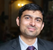# QlikView App Dev

Discussion Board for collaboration related to QlikView App Development.

Announcements
Skip the ticket, Chat with Qlik Support instead for instant assistance.
cancel
Showing results for
Did you mean:Partner - Creator II

## Set Analysis confusion

Hi Experts.

I am having a weird experience with set analysis. Here are my expressions.

=sum({<MONTH={'01/02/2016'}>} DATA)

=sum({<MONTH={"=\$(vL.AMaxMon)"}>} DATA)

where

=\$(vL.AMaxMon) is max({<DATA_STATUS={'ACTUAL'}>}MONTH) which evaluates to  01/02/2016

That should be the same as sum({<MONTH={'01/02/2016'}>} DATA) but I see different results of sum.

Are there  limitations of some kind on set analysis.

I am showing this is text boxes. Can some one help??

1 Solution

Accepted SolutionsMVP

Can you try this:

=Sum({<MONTH={'\$(=\$(vL.AMaxMon))'}>} DATA)

or this

=Sum({<MONTH={'\$(=vL.AMaxMon)'}>} DATA)

5 RepliesMVP

Can you try this:

=Sum({<MONTH={'\$(=\$(vL.AMaxMon))'}>} DATA)

or this

=Sum({<MONTH={'\$(=vL.AMaxMon)'}>} DATA)Partner - Creator II
Author

Thanks for prompt suggestion Sunny

=Sum({<MONTH={'\$(=vL.AMaxMon)'}>} DATA)

gives

Error: Error in set modifier ad hoc element list:

',' or ')' expected

But

=Sum({<MONTH={'\$(=\$(vL.AMaxMon))'}>} DATA)

works can you explain why?MVP

I guess we need to use something that can replace 01/02/2016 in the set analysis. Whatever that thing is, need to be expanded using \$(=...). My guess is that if you put \$(vL.AMaxMon) in a text box, it would expand to this -> 01/02/2016.

So this is what I usually do. Whatever I need in my set analysis, I create that in the text box object and then copy the whole thing and paste it into my dollar sign expansion. Does that make sense?Partner - Creator II
Author

Thanks A Million stalwar1MVP

Not a problem, I am glad I was able to helpCommunity Browser